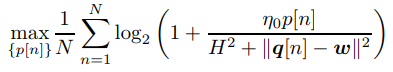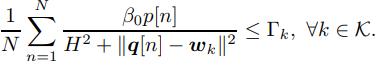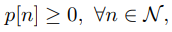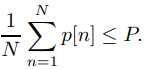# CVX ignoring constraints and returning Inaccurate/Solved

Hello, I am trying to solve an optimization problem as shown belowsubject to the following constraints:where p[n] is power being transferred in a cognitive wireless communications network, and gamma outlines a predetermined interference limit which needs to be minimized. I have written the following code in CVX to model the problem above:

``````K = 2;  %Number of primary users
T = 200; %Mission time period
f_s = 1; %sample frequency
N = T/f_s; %Number of timesteps
delta_t = 1/f_s; %sample period
H = 100;    %Altitude
b_0 = 10^-3;    %Beta naught channel power gain
q_i = [-1000 1000]; %initial UAV pos
q_f = [1000 -1000]; %final UAV pos
sigma_squared = 10^-8; %Sigma squared SNR level
q_n = zeros(N,2); %vector space for optimized trajectory
qj_n = zeros(N,2);  %Previous iteration trajectory
for i = 1:N %designing straight line trajectory
qj_n(i,1) = ((2000/(N-1))*(i-1))-1000;
qj_n(i,2) = 1000 - ((2000/(N-1))*(i-1));
end
qj_n(N,1) = q_f(1,1);
qj_n(N,2) = q_f(1,2);
p_ave = 0; %average optimized power
w_sr = [0 0]; %Secondary user position
P = 1; % power threshold
gamma_k = 10^-12; % interference threshold
w_k = [-500 500; 500 -500]; %position of primary users
p_n = zeros(N,1); %vector for optimized power value at p(i)
cvx_clear;
fprintf('Optimization program has started \n');
[p_n, p_ave] = p_opt(K, N, H, b_0, sigma_squared, qj_n, w_sr, P, gamma_k, w_k);
fprintf('The optimized power is %d\n', p_ave);

function [p_optimized, p_rate] = p_opt(K, N, H, b_0, sigma_squared, qj_n, w_sr, P, gamma_k, w_k)
cvx_begin;
n_0 = b_0/sigma_squared;
p_optimized = cvx(zeros(N,1)); %vector for optimized power value at p(i)
int_k = cvx(zeros(K,1)); %interference to each primary user at position q(i)
p_sum = 0;
variable x(N,1)
for i=1:N %finding sum of optimized power
p_sum = p_sum + (-rel_entr(1, 1 + ((n_0 * x(i,1))/(H^2 + sum_square(qj_n(i,:) - w_sr)))))/log(2);
p_optimized(i,1) = x(i,1);
end
p_rate = p_sum/N;
maximize (p_rate);
subject to
for i=1:N %power cannot be negative
x(i,1) >= 0;
%     x(i) <= 2;
end
(sum (x))/N <= P; %average power cannot be above the threshold
for i=1: K %interference constraint
int_p = 0;
for j=1:N
int_p = int_p + ((b_0*x(j,1))/(H^2 + sum_square(qj_n(j,:)-w_k(i,:))));

end
int_k(i,1) = int_p/N;
int_k(i,1) <= gamma_k;

end
cvx_end;
end
``````

When running this CVX ignores the interference constraint (gives solved interference of 0.35x10^8 when it needs to be below 10^12) and results in an inaccurate/solved status. It would seem intuitive that if all values of power were simply scaled down to a lower value then you could satisfy the interference constraint, but CVX does not seem to want to do this.

Any advice on why this may be happening would be appreciated. Thank you.

Let me break the bad news to you.

If you have a problem where the norm of the solution is 10^12 you have an unstable problem. That can be proven mathematically. Standard optimizers such as sedumi, Mosek is likely to have hard time to produce accurate results.

Building a better model is likely to be the only answer.

1 Like

Hi,

If the model itself is the reason that I am receiving such a result what changes should be made to the model in order to get an accurate solution?

Scaling etc.?

Thank you

``````Status: Inaccurate/Solved
Optimal value (cvx_optval): +0.925786

>> disp(int_k)
1.0e-08 *

0.357410163769755
0.357410163769665

>> disp(gamma_k)
1.000000000000000e-12
``````

The constraint `int_k <= gamma_k` is satisfied within solver tolerance. You could potentially specify a somewhat tighter feasibility tolerance using `cvx_solver_settings` http://cvxr.com/cvx/doc/solver.html#advanced-solver-settings , but that might not necessarily work very well, and I woulldn’t advise doing that with SDPT3.

But as @Erling write, if the distinction between 1e-8 and 1e-12 is important to you, then you need to improve your model. Or use a higher precision (e.g., quad precision) solver, which can not be done under CVX.